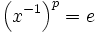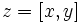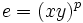# Omega-1 of odd-order class two p-group has prime exponent

This article states and (possibly) proves a fact that is true for odd-order p-groups: groups of prime power order where the underlying prime is odd. The statement is false, in general, for groups whose order is a power of two.
View other such facts for p-groups|View other such facts for finite groups

## Statement

Let$P$ be a p-group (not necessarily finite) of nilpotence class two, where$p$ is an odd prime. Then, the subgroup:$\Omega_1(P) = \langle x \in P \mid x^p = e \rangle$

is a group of prime exponent: in other words, every non-identity element in$\Omega_1(P)$ has order$p$. Equivalently, in a$p$-group of nilpotence class two, the product of any two elements of order$p$ is either trivial, or has order$p$.

## Related facts

### Breakdown for the prime two

Further information: dihedral group:D8

The result does not hold when$p = 2$, a counterexample is the dihedral group of order eight, which is equal to its$\Omega_1$, even though the exponent is$4$.

### Breakdown for higher nilpotence class

Further information: wreath product of groups of order p

For any prime$p$, we can construct a$p$-group generated by elements of order$p$, and with exponent$p^2$. This is the wreath product of groups of order$p$; equivalently, it is the$p$-Sylow subgroup of the symmetric group on$p^2$ elements.

It is particularly easy to see from the latter description that the exponent is$p^2$: there is a cycle of length$p^2$ in the symmetric group, and by Sylow's theorem, some conjugate of this cycle must lie in any Sylow$p$-subgroup. Further, an examination of cycle decompositions shows that there is no element of order$p^3$.

Further, we can construct a$p$-group generated by elements of order$p$, with exponent$p^r$ for any$r$. This is the iterated wreath product of the cyclic group of order$p$ with itself$r$ times; equivalently, it is the$p$-Sylow subgroup of the symmetric group on$p^r$ elements. This subgroup contains a cycle of length$p^r$, and hence, the exponent must be at least$p^r$.

## Proof

It suffices to show that the set of elements$x$ such that$x^p = e$, is a subgroup.

Given: A$p$-group$P$ of nilpotence class two, where$p$ is an odd prime.

To prove: The set of$x \in P$ such that$x^p = e$ is a subgroup

Proof: Clearly, the identity element is in this set, and if$x^p = e$ then$\left(x^{-1}\right)^p = e$, so the set is closed under inversion. Thus, what we need to show is that:$x^p = e, y^p = e \implies (xy)^p = e$

Let's prove this. Suppose$z = [x,y]$ denotes the commutator of$x$ and$y$. Then, since$G$ has nilpotence class two,$z$ commutes both with$x$ and with$y$. Thus, we have:$xy = (yx)z$

And further:$x^2y^2 = xxyy = x(yx)zy = (xy)^2z$

By induction, we can show that:$x^jy^j = (xy)^jz^{j(j-1)/2}$

Setting$j = p$, we get:$e = (xy)^pz^{p(p-1)/2}$

We also have the identity:$z^p = [x,y]^p = [x,y^p] = [x,e] = e$

So if$p \ne 2$, then$p | p(p-1)/2$, and so$z^{p(p-1)/2} = e$, and we get:$e = (xy)^p$

as desired.